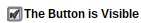Direction Fields - Maple Help

Home : Support : Online Help : Math Apps : Calculus : Differential : Direction Fields

Direction Fields

 Main Concept A differential equation (DE) is a mathematical equation which relates an unknown function and one or more of its derivatives. DEs are an important tool in many areas of pure and applied mathematics, as well as other subjects such as physics, engineering, economics, and biology. The order of a DE is the order of the highest derivative of the dependent variable with respect to the independent variable appearing in the equation.   The focus of this app is on first-order differential equations, which only include the first derivative and the function itself. These DEs often have the form  where x is the independent variable, y is the dependent variable, and so  , also written, is the derivative of the function $y\left(x\right)$ with respect to x.   Although there are many techniques for solving first-order DEs, it can be very difficult or even impossible to solve them explicitly in some cases, and so there is need for a way to visualize and numerically approximate the solution curves. This is where direction fields become useful.   A direction field (or slope field) is a graphical representation of the solutions of a first-order differential equation achieved without solving the DE explicitly. At each point (x,y) in the plane, you plot the direction vector $\left[\begin{array}{c}1\\ f\left(x,y\right)\end{array}\right]$, which is tangent to (has the same slope as) the solution curve through that point. These vectors are often normalized to be of consistent length to emphasize their direction rather than magnitude. You can then approximate the solution curves by following the direction vectors.   Often, you are also given an initial condition,  . This allows you to illustrate a specific curve in the family of solutions by ensuring that the curve you sketch passes through the point  while still following in the directions of the vectors.

Choose a first-order differential equation from the list or enter your own in the text box below the list to plot its direction field. Enter values for ${x}_{0}$ and ${y}_{0}$ in the text boxes below the plot to set an initial condition, then select Add a Solution Curve to add the solution curve which satisfies this initial condition.

Click the plot twice to define the opposite corners of a rectangular viewing area into which you would like to zoom. To zoom out from this region, click Zoom Out, or click Default Zoom to return to the  viewing window you started with.

Warning: Due to the numerical methods being used to approximate the solution curve, the curve may end abruptly when approaching an asymptote.

 = yxx+yx^2 - 3*x +1cos(x)1/(1 + exp(-x))x*sin(y)(y^2)*xsin(x + y)Custom DEInitial Condition: $y($